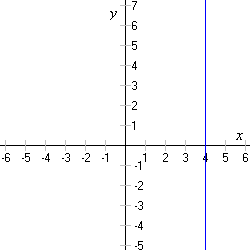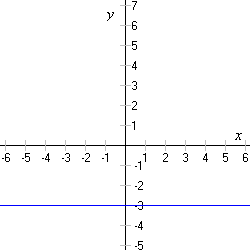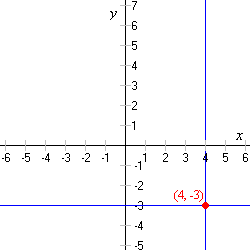# Horizontal and Vertical Lines

Equations of horizontal and vertical lines only have one variable . The equation $x=4$ represents a vertical line which crosses the $x$ -axis at the point $\left(4,0\right)$ . Every ordered pair with $4$ as its first coordinate is a solution . (The equation means “ $x$ is equal to $4$ , and $y$ can be whatever it wants.”)Similarly, the equation

$y=-3$

is a horizontal line which crosses the $y$ -axis at $\left(0,-3\right)$ .The point where the horizontal and vertical lines intersect is also easy to find.